# How to Add Period After Numbers List in Excel?

A period is nothing but a dot(.) symbol which we can use after each number in the list of numbers. A period is used in Excel to generally represent that a number has ended. Adding a period can be done in two ways in Excel. The first one is by using the formula and the other by using the Format option. Read through this tutorial to find out how you can add period marks after numbers list in Excel in a simple and efficient manner.

## Adding Period After Numbers List using Formula

Let us take a simple example to understand how period marks can be added after a Numbers list using formulas.

### Step 1

Let us assume we have an Excel sheet that contains the list of numbers similar to the screenshot shown image −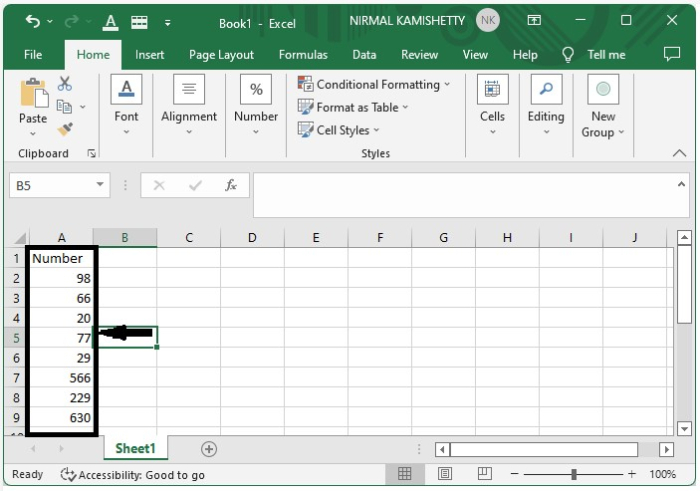To add Period marks, click an empty cell and enter the formula =A1&"." as in the formula box and press the Enter button to get the first result, as shown in the following screenshot −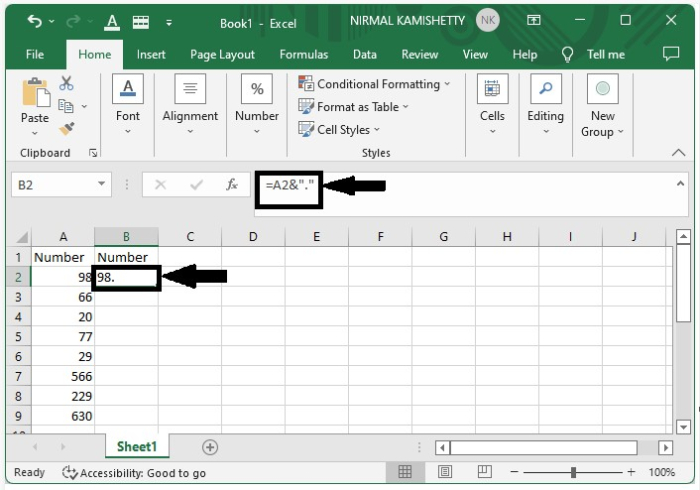### Step 2

We can get all the other results just by dragging down from the first result till all the results are filled. The final output screen will appear as follows −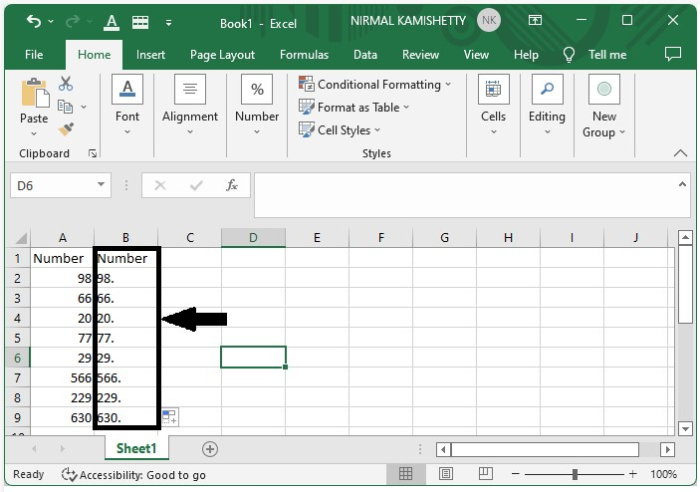## How to Add Period after Numbers List using Format Cells Option

Let us take another example to show how you can add period marks after numbers list using "Format Cells" option.

### Step 1

Let us consider the same data which we used in the above example.

Now select the data, right-click on it, and select "Format Cells". It will open a new pop-up window, as shown below −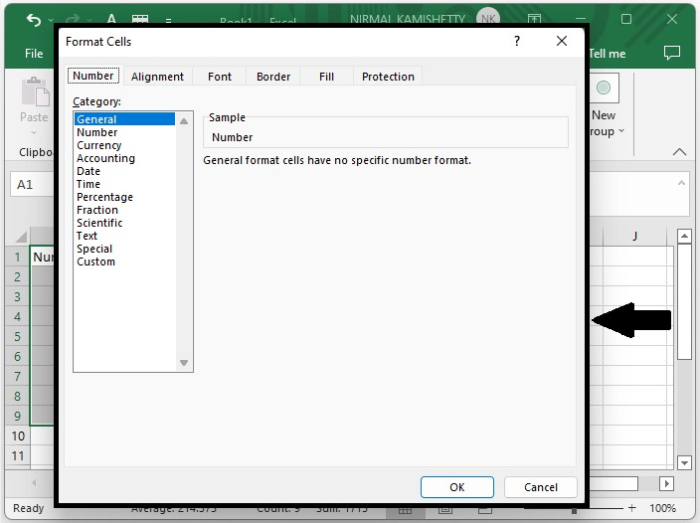### Step 2

Now, click "Custom" and enter the "Type" as "#" and press "OK" to get the final result. The screen will appear as the one shown below −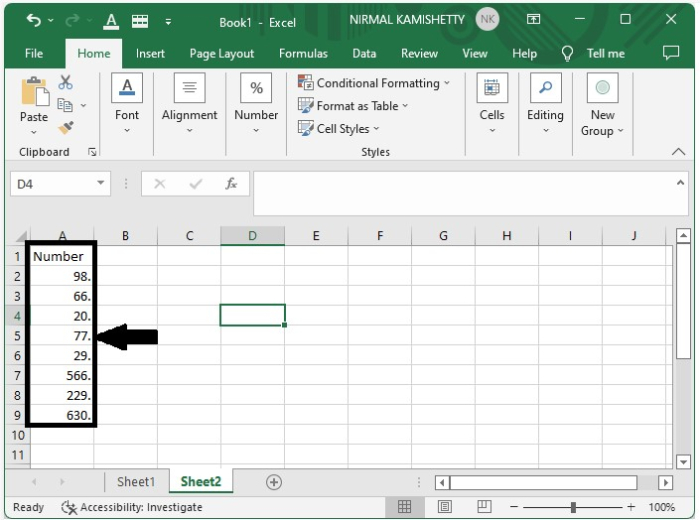## Conclusion

In this tutorial, we explained two simple and efficient ways that you can use to add period marks after numbers list in Excel.